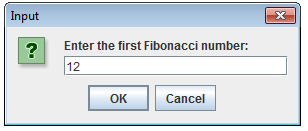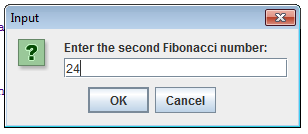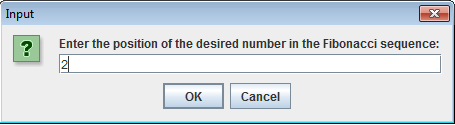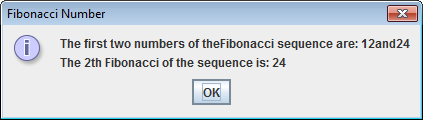# Fibonacci Number in Java

The following Java program application is a Fibonacci number. I will be using the JCreator IDE in developing the program. To start in this tutorial, first open the JCreator IDE, click new and paste the following code
`import javax.swing.JOptionPane; public class FibonacciNumber{	public static void main (String[] args)	{		//declaration of variables		String inputString;		String outputString;		int previous1;		int previous2;		int current = 0;		int counter;		int nthFibonacci; 		inputString = 			JOptionPane.showInputDialog("Enter the first Fibonacci number: ");			previous1 = Integer.parseInt(inputString); 		inputString = 			JOptionPane.showInputDialog("Enter the second Fibonacci number: ");			previous2 = Integer.parseInt(inputString); 		outputString = "The first two numbers of the"				+ "Fibonacci sequence are: "					+ previous1 + "and" + previous2;		inputString = 			JOptionPane.showInputDialog("Enter the position of the desired "				+ "number in the Fibonacci sequence: ");		nthFibonacci = Integer.parseInt(inputString); 		if (nthFibonacci == 1)    //copies the value of previous1 into current			current = previous1;			else if (nthFibonacci == 2)//copies the value of previous2 to current				current = previous2;		else    //calculate the desired Fibonacci number		{			counter = 3;			while (counter <= nthFibonacci)		{			current = previous2 + previous1;			previous1 = previous2;			previous2 = current;			counter++; 			}		}		outputString = outputString + "\nThe "				+ nthFibonacci				+ "th Fibonacci of "				+ "the sequence is: "					+ current; 			JOptionPane.showMessageDialog(null, outputString,						"Fibonacci Number",						JOptionPane.INFORMATION_MESSAGE);				System.exit(0);	}}`
Sample Run:The program works as follow: The statement
` inputString = 			JOptionPane.showInputDialog("Enter the first Fibonacci number: ");			previous1 = Integer.parseInt(inputString); `
prompts the user for the first Fibonacci number that is, `previous1` and it stores into `inputString`. The statement
` inputString = 			JOptionPane.showInputDialog("Enter the second Fibonacci number: ");			previous2 = Integer.parseInt(inputString);`
display the input dialog box to prompt the user for the second Fibonacci number. The statement
` outputString = "The first two numbers of the"				+ "Fibonacci sequence are: "					+ previous1 + "and" + previous2;`
create output and append previous1 and previous2. The statement
` inputString = 			JOptionPane.showInputDialog("Enter the position of the desired "				+ "number in the Fibonacci sequence: "); 		nthFibonacci = Integer.parseInt(inputString);`
displays the input dialog box to prompt the user for the desired Fibonacci number and store the nth Fibonacci into inputString. The statement
` JOptionPane.showMessageDialog(null, outputString,						"Fibonacci Number",						JOptionPane.INFORMATION_MESSAGE);`
display the output dialog box showing the first two and nth Fibonacci number.